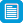# Search results

## Refine by topic

Main topic Specific topic Related topic
Listed under:  Mathematics  >  Shapes (Geometry)  >  Quadrilaterals### reSolve: Geometry: Trapezium Pieces

This lesson explores different shapes that can be formed by cutting a trapezium in two with one straight line. Students are challenged to classify and name the shapes that are made, and justify their classifications based on the definitions and properties of shapes. The lesson is outlined in detail including curriculum ...### reSolve: Polygon Pieces

This lesson explores the geometry of cutting polygons in different ways and using algebra to express subsequent findings. Students use one straight cut to divide a convex polygon into two new polygons. They make generalisations about the total number of sides of the two new polygons, and about the number of different combinations ...### TIMES Module 13: Measurement and Geometry: construction - teacher guide

This is a 30-page guide for teachers that explains the central role of construction and presents examples of constructions.### Geometric reasoning - congruence

This is a website designed for both teachers and students that introduces congruence of shapes in the plane through transformations. In particular, transformations, translations, reflections in an axis and rotations of multiples of 90 degrees are used to define congruence and to identify congruent shapes. The four congruence ...### Laptop wrap: Geometry gems

A page with a focus on using GeoGebra to enhance student understanding of various geometric properties and the importance of reasoning in proof. A laptop-friendly resource that includes supporting activities and links to resources.This series of six lessons explores geometry using real world contexts focussed on the dynamics of linkages and moving joints of everyday tools and objects. Students use physical models and computer simulations, the lessons move from a view of geometry as a study static diagrams to encompass movement. Each lesson is outlined ...### Tessellating tiles

In this teaching resource students learn how to construct shapes that will tessellate (or tile) a plane area. Starting with a regular shape known to tessellate (square, equilateral triangle, hexagon), students apply geometrical transformations to the sides of the shape to create new shapes that tessellate. There are links ...### TIMES Module 20: Measurement and Geometry: parallelograms and rectangles - teacher guide

This is a 17-page guide for teachers. It contains the definitions, properties and tests for parallelograms and rectangles. Proofs of the properties and tests are given. Constructions for parallelograms and rectangles are given.